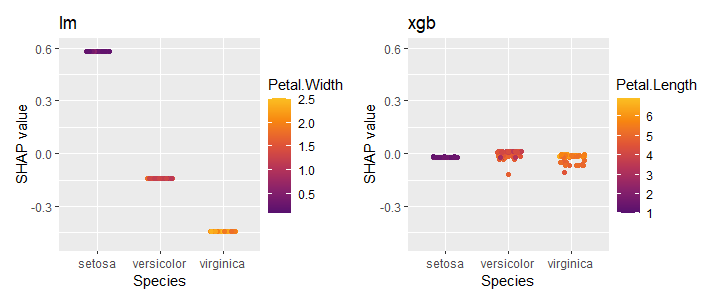# Multiple ‘shapviz’ objects

## Overview

Sometimes, you will find it necessary to work with several “shapviz” objects at the same time:

• To visualize SHAP values of a multiclass or multi-output model.
• To compare SHAP plots of different models.
• To compare SHAP plots between subgroups.

To simplify the workflow, {shapviz} introduces the “mshapviz” object (“m” like “multi”). You can create it in different ways:

• Use shapviz() on multiclass XGBoost or LightGBM models.
• Use shapviz() on “kernelshap” objects created from multiclass/multioutput models.
• Use c(Mod_1 = s1, Mod_2 = s2, ...) on “shapviz” objects s1, s2, …
• Or mshapviz(list(Mod_1 = s1, Mod_2 = s2, ...))

The sv_*() functions use the {patchwork} package to glue the individual plots together. An exception is sv_importance(..., kind = "bar"), which produces a dodged barplot via {ggplot2}, by default.

## Example: Multiclass XGBoost model

library(shapviz)
library(ggplot2)
library(patchwork)
library(xgboost)

params <- list(objective = "multi:softprob", num_class = 3, nthread = 1)
X_pred <- data.matrix(iris[, -5])
dtrain <- xgb.DMatrix(X_pred, label = as.integer(iris[, 5]) - 1)
fit <- xgb.train(params = params, data = dtrain, nrounds = 50)

# Create "mshapviz" object (logit scale)
(x <- shapviz(fit, X_pred = X_pred, X = iris))
#> 'mshapviz' object representing 3 'shapviz' objects:
#>    'Class_1': 150 x 4 SHAP matrix
#>    'Class_2': 150 x 4 SHAP matrix
#>    'Class_3': 150 x 4 SHAP matrix

# Contains "shapviz" objects for all classes
all.equal(x[], shapviz(fit, X_pred = X_pred, X = iris, which_class = 3))
#>  TRUE

# Better names
names(x) <- levels(iris$Species) x #> 'mshapviz' object representing 3 'shapviz' objects: #> 'setosa': 150 x 4 SHAP matrix #> 'versicolor': 150 x 4 SHAP matrix #> 'virginica': 150 x 4 SHAP matrix # SHAP plots sv_importance(x)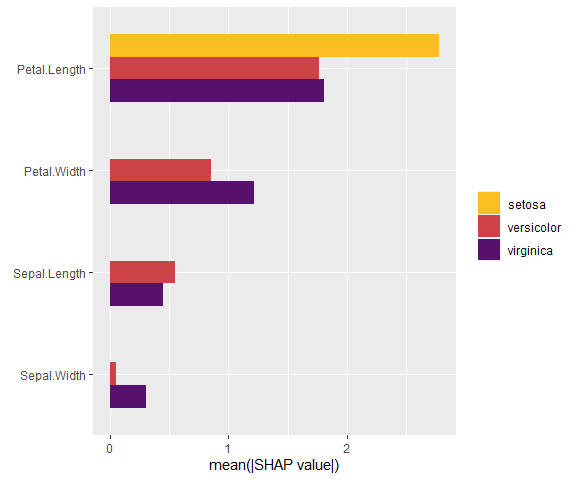sv_importance(x, bar_type = "stack") # Same but stacked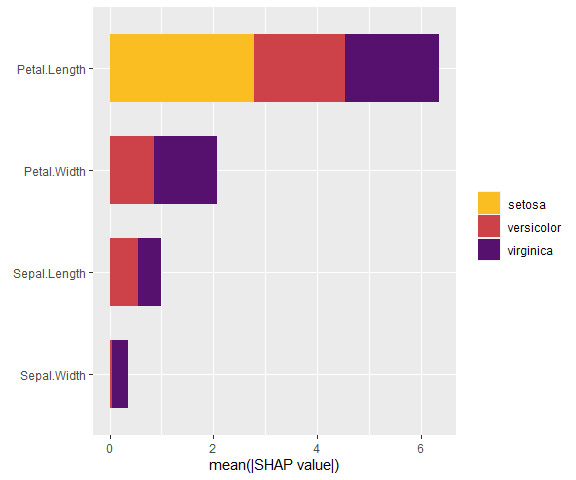sv_dependence(x, v = "Petal.Length") + plot_layout(ncol = 1) & ylim(-3, 4) # Same y scale to get impression on strength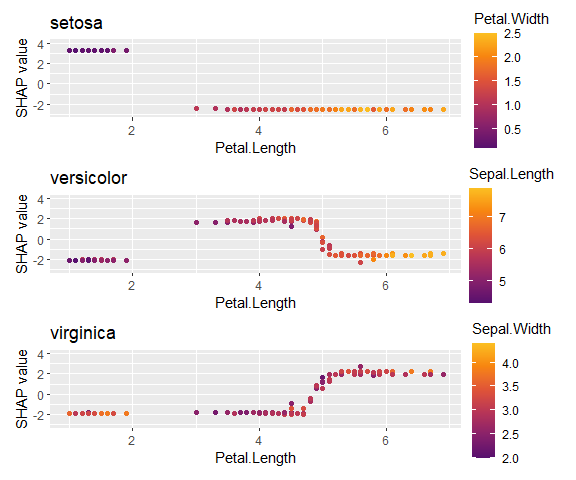### Similar for LightGBM (only code) library(shapviz) library(lightgbm) # Model params <- list(objective = "multiclass", num_class = 3) X_pred <- data.matrix(iris[, -5]) dtrain <- lgb.Dataset(X_pred, label = as.integer(iris[, 5]) - 1) fit <- lgb.train(params = params, data = dtrain, nrounds = 50) x <- shapviz(fit, X_pred = X_pred, X = iris) sv_importance(x) ### Or for random forest with {kernelshap} Since Kernel SHAP is model agnostic, we can get SHAP values on probability scale. library(shapviz) library(kernelshap) library(ggplot2) library(patchwork) library(ranger) # Model fit <- ranger(Species ~ ., data = iris, num.trees = 100, probability = TRUE) # "mshapviz" object x <- kernelshap(fit, X = iris[-5], bg_X = iris) shp <- setNames(shapviz(x), levels(iris$Species))
# all.equal(shp[], shapviz(x, which_class = 3))

sv_importance(shp)

sv_dependence(shp, v = "Sepal.Width") +
plot_layout(ncol = 2) &
ylim(-0.03, 0.035)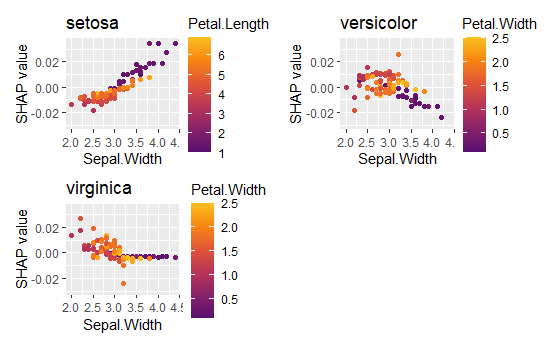## Example: SHAP subgroup analysis

Let’s compare SHAP dependence plots across Species.

library(shapviz)
library(ggplot2)
library(patchwork)
library(xgboost)

X_pred <- data.matrix(iris[, -1])
dtrain <- xgb.DMatrix(X_pred, label = iris[, 1])
fit_xgb <- xgb.train(params = list(nthread = 1), data = dtrain, nrounds = 50)

# Create "mshapviz" object
shap_xgb <- shapviz(fit_xgb, X_pred = X_pred, X = iris)
x_subgroups <- split(shap_xgb, f = iris\$Species)

# SHAP analysis
sv_importance(x_subgroups, bar_type = "stack")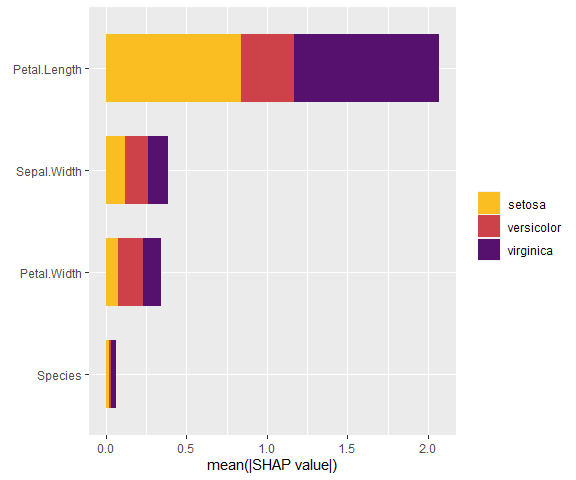sv_dependence(x_subgroups, v = "Petal.Length") +
plot_layout(ncol = 1) &
xlim(1, 7) &
ylim(-1.4, 2.2)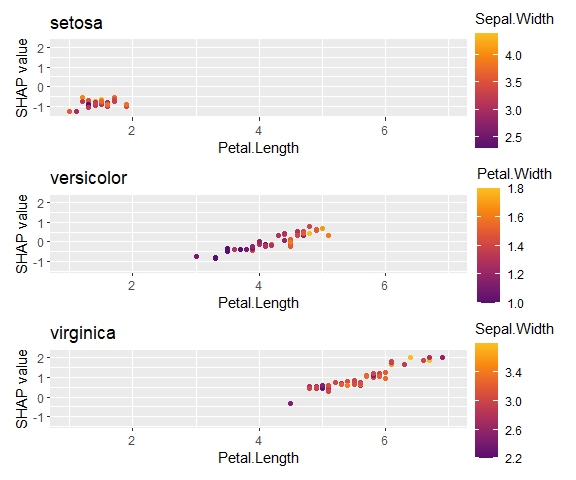## Example: Different models

In the last example, we used a regression model fitted via XGBoost. How does it compare with a linear regression?

### Fit linear regression and use {kernelshap} to get SHAP values

library(kernelshap)

fit_lm <- lm(Sepal.Length ~ ., data = iris)
shap_lm <- shapviz(kernelshap(fit_lm, iris[-1], bg_X = iris))

# Combine "shapviz" objects
mshap <- c(lm = shap_lm, xgb = shap_xgb)
mshap

#> 'mshapviz' object representing 2 'shapviz' objects:
#>   'lm': 150 x 4 SHAP matrix
#>   'xgb': 150 x 4 SHAP matrix

# SHAP analysis
sv_importance(mshap)

sv_dependence(mshap, v = "Species") &
ylim(-0.5, 0.6)